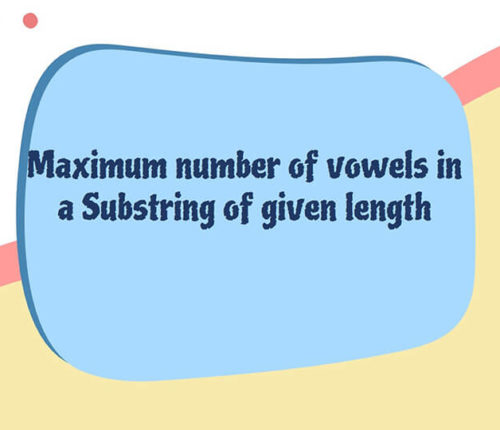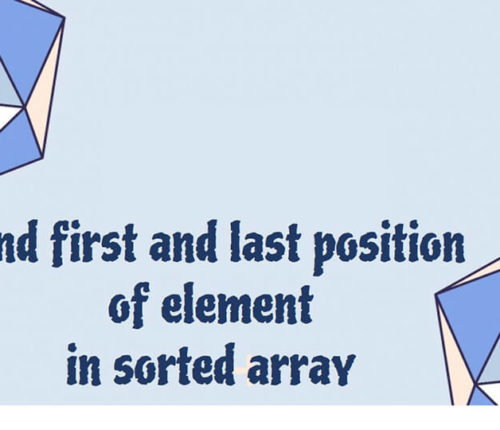# Find the local minima in array

If you want to practice data structure and algorithm programs, you can go through Java coding interview questions.

In this post, we will see how to find the local minima in the array.

## Problem

An element is local minima if it is less than its neighbors.

int [] arr = {10, 5, 3, 6, 13, 16, 7};
Output: 2

int []arr = {11,12,13,14};
Output: 11

int []arr = {10};
Output: 10

int []arr = {8,6};
Output: 6

## Solution

### Naive approach

You can use for loop and compare neighbor elements, you will get the local minima.
Worst case time complexity will be `o(n)`.

### Efficient approach

You can use binary search to find local minima.

Worst case time complexity will be `o(log n)`.
Here is simple algorithm

• Find the `mid` element
• If `mid` element is `less` than both the neighbor then return it.
• If `mid` element is `greater` than its left neighbor, then go left
• else go right.

When you run above program, you will get below output.

Local Minima is: 3

That’s all about how to find the local minima in a array

import_contacts

### You may also like:## Related Posts

•18 June

### Maximum Number of Vowels in a Substring of Given Length

Table of ContentsApproach – 1 Generate All Substrings Using substring() MethodApproach – 2 Using Sliding Window Method (Linear Time Solution) In this article, we will look at an interesting problem related to the Strings and [Sliding-Window Algorithm](https://java2blog.com/sliding-window-maximum-java/ “Sliding-Window Algorithm”). The problem is : "Given a String we have to Find the Maximum Number of Vowel […]

•04 June

### Search for a range Leetcode – Find first and last position of element in sorted array

Table of ContentsApproach 1 (Using Linear Search)Approach 2 (Using Modified Binary Search-Optimal) In this article, we will look into an interesting problem asked in Coding Interviews related to Searching Algorithms. The problem is: Given a Sorted Array, we need to find the first and last position of an element in Sorted array. This problem is […]

•30 April

### Convert Postfix to Infix in Java

Learn about how to convert Postfix to Infix in java.

•30 April

### Convert Prefix to Postfix in Java

Learn about how to convert Prefix to Postfix in java.

•16 April

### Data Structures in java

•